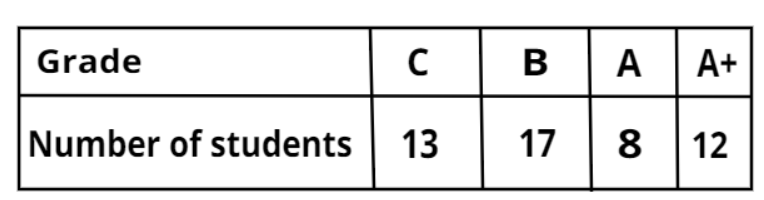Questions & AnswersQuestionAnswers

# The grade marks of 50 students of a class are recorded as belowA student of the class is selected at random, what is the probability that the selected student has obtained grade A?${\text{A}}{\text{. }}\dfrac{4}{{25}} \\ {\text{B}}{\text{. }}\dfrac{{16}}{{50}} \\ {\text{C}}{\text{. }}\dfrac{4}{{50}} \\ {\text{D}}{\text{. }}\dfrac{8}{{25}} \\$Answer Verified
Hint- Here, we will be using the general formula for the probability of occurrence of an event.

Given, Total number of students in a class$= 50$
According to the given data in the table, we can say that
Number of students having grade C$= 13$, Number of students having grade B$= 17$, Number of students having grade A$= 8$ and Number of students having grade A+$= 12$
As we know that the general formula for probability is given by
Probability of occurrence of an event$= \dfrac{{{\text{Number of favourable outcomes}}}}{{{\text{Total number of possible outcomes}}}}$
Here, the favourable event is that the selected student has obtained grade A.
So, Number of favourable outcomes$=$Number of students who has obtained grade A$= 8$
Total number of possible outcomes$=$Total number of students in a class$= 50$
Therefore, Required probability$= \dfrac{8}{{50}} = \dfrac{4}{{25}}$
Hence, the probability that the selected student has obtained grade A is $\dfrac{4}{{25}}$.
Option A is correct.

Note- In these types of problems, the favourable event is the event whose probability is asked to find out. In this particular problem, extra data is also provided in the question itself (number of students who have obtained grades C, B and A+) which is not being used anywhere to get to the answer.
Bookmark added to your notes.
View Notes
Table of 50 - Multiplication Table of 50How to Calculate the Percentage of Marks?Tally MarksWhat are the Domains of the EarthWhat are the Challenges of Democracy?Marks Wise CBSE Important QuestionsFailures are The Pillars of Success EssayQuotation MarksWhat are the Functions of the Human Skeletal System?Important 3 Marks Question For CBSE Class 10 Maths